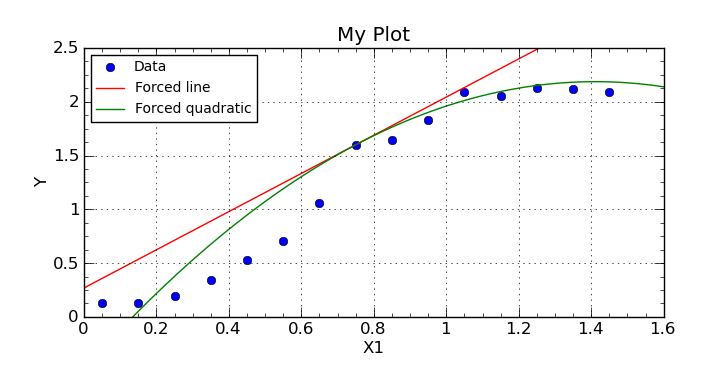Forcing a curve fit through a specific point

# Forcing a curve fit through a specific point

If you want to force a particular curve fit through a specific point, you can do so via the advanced models in CurveExpert Pro (you can actually do it via a one-line equation as well, but the result is a little ugly). Forcing through a point acknowledges that you lose one parameter in your fit; meaning that one parameter must obey a specific relationship given the other np-1 parameters. So, just solve for the one and compute it appropriately in the model.

For example, if you want to force a line through a given point (,), we have , so upon rearranging, . There is only one free parameter left out of the two, which is . Implementing this condition in an advanced user model looks like this (some comments removed for brevity):

[python]
name = "Forced line"
equation = "a*x + b"
latexequation = r"a x + \tilde b"
nindvar = 1

def evaluate(x,a):
xf = 0.75
yf = 1.6
btilde = yf - a*xf
return a*x + btilde

def initialize(x,y):
return 1.0,

[/python]

Doing the same thing with a quadratic, we have , so upon rearranging, . Thus, we only have two free parameters and . Implementing this condition in an advanced user model looks like this:

[python]